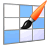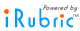find rubric
 delete .rubinfoitem { padding:5px; white-space: nowrap; } Adding Fractions  The students have been working on all the concepts leading up to adding fractions. Comparing fractions with unlike denominators, making equivalent fractions. Rubric Code: T23BW75 Ready to use Public Rubric Subject: Math   Type: Assessment   Grade Levels: K-5, 6-8Adding Fractions Beginning 1 pts Developing 2 pts Accomplished 3 pts Exemplary 4 pts Find LCM of Denominators Beginning With intensive assistance, the student somewhat understands the concept of listing the multiples of the denominators, but their work is not complete. Developing With some assistance, the student somewhat understands the concept of listing the multiples of the denominators, but their work is not complete. Accomplished With minimal assistance, the student understands and lists the multiples of the denominators, showing the LCM by circling it, or making it the fractions new denominator. Exemplary The student fully understands and lists the multiples of the denominators, showing the LCM by circling it, or making it the fractions new denominator. Make Equivalent Fractions Beginning With intensive assistance, the student somewhat understands how change the fractions to equivalent fractions with the same denominators. Developing With some assistance, the student somewhat understands the concept of making equivalent fraction, but their understanding is not complete. They make may the new denominator the LCM but is not sure about how to get the new numerator. Accomplished With minimal assistance the student is able to make equivalent fractions from making the LCM of the unlike denominators the new denominators and then multiples the numerator by the same number they multiplied their old denominator by to get their new denominator. Exemplary The student is able to make equivalent fractions from making the LCM of the unlike denominators the new denominators and then multiples the numerator by the same number they multiplied their old denominator by to get their new denominator. Add the Fractions Beginning The student does not know how to correctly add or subtract the fractions with unlike denominators without assistance. Developing With some assistance the student somewhat understands that when the denominator is different, they need to make equivalent fractions. They make an attempt to find the new numerators/denominators and adds or subtracts only the numerator. Accomplished With minimal assistance the student somewhat understands that when the denominator is different, they need to make equivalent fractions. They make an attempt to find the new numerators/denominators and adds or subtracts only the numerator. Exemplary The student fully understands to add the new numerators together, while the denominator stays the same, as well as adding the whole numbers. Convert Mixed Numbers to Improper Fractions Beginning The student does not know or does not convert mixed numbers into improper fractions without assistance. Developing With some assistance the student may somewhat understand to convert mixed numbers into improper fractions. An attempt is made to convert mixed numbers into improper fractions. Accomplished With minimal assistance the student somewhat understands that when there is a mixed number, they need to multiply the denominator by the natural number, add the product to the numerator to come up with the improper fraction. Exemplary The student is able to convert mixed numbers to improper fractions by multiplying the denominator by the natural number, adding the product to the numerator to come up with the improper fraction.

Keywords:

Subjects:

Types:

You may also be interested in:

Do more with this rubric:

Preview this rubric.

Modify this rubric.

Make a copy of this rubric and begin editing the copy.

Show a printable version of this rubric.

Add this rubric to multiple categories.

Bookmark this rubric for future reference.
Assess

Test this rubric or perform an ad-hoc assessment.

Build a gradebook to assess students.

Apply this rubric to any object and invite others to assess.
Share

Email this rubric to a friend.

Discuss this rubric with other members.

Do more with rubrics than ever imagined possible.

Only with iRubrictm.